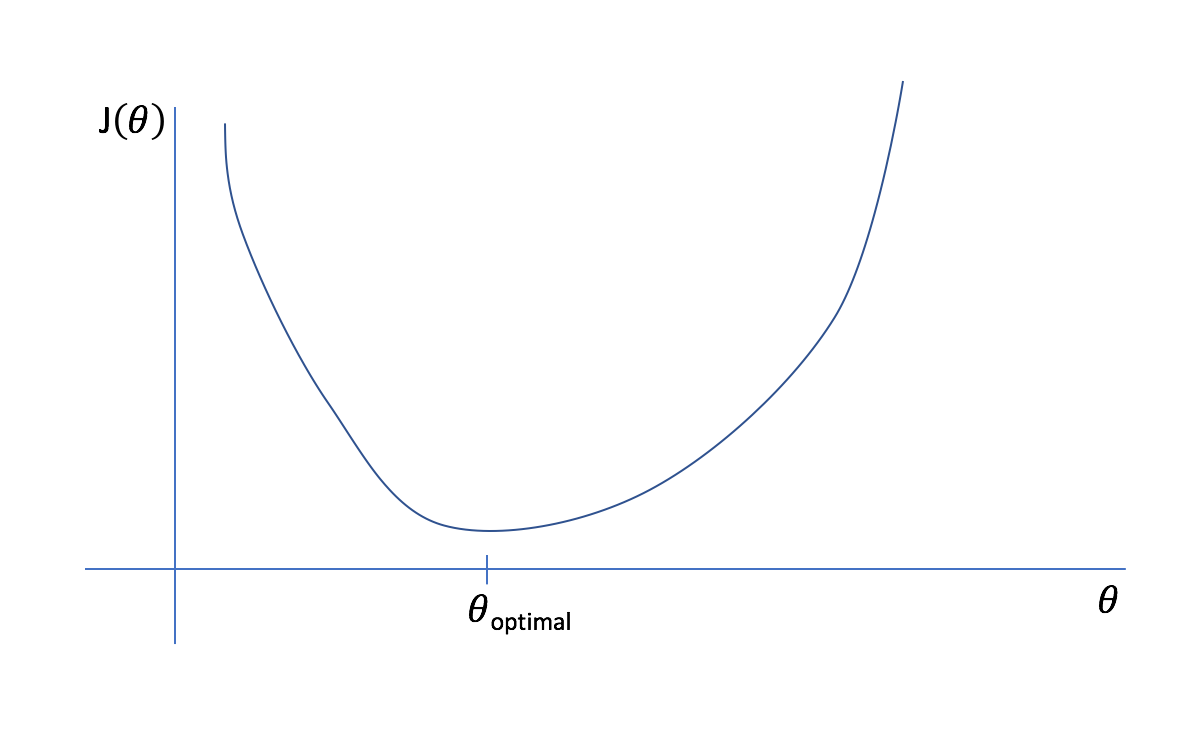# Simplifying Optimization : A Study of AdaGrad

Let us begin to grasp the concept of AdaGrad with a brief overview of one of the most important optimization algorithms in deep learning — Gradient Descent. It will be in regulating the hyper-parameters of Gradient Descent that we will utilize the concept of AdaGrad or Adaptive Gradient Method.A plot of Cost Function J( ϴ) vs ϴ

Gradient Descent, in short, is the procedure of finding the right set of values of ϴ (i.e ϴ optimal) to minimize the cost function J(ϴ) .

### θt+1 = θt — α(∂L∕∂θt)

Thus as is seen in the above equation, based on α and the gradients (∂L∕∂θt) we take steps in updating our parameter θt+1 to gently reach the global optimum θoptimal .

In these gifs it is evident that the learning rate plays a huge role in determining how well the algorithm shall converge, when it will converge and whether it will diverge on reaching the minima.

It will be based on this learning rate that we will base our understanding of AdaGrad and it’s place within Gradient Descent.

Without using any optimization algorithm, like AdaGrad or Adam or RMSProp, the learning rate stays the same through every iteration. But looking at the two learning rates up top, it seems that while α = 0.05 is diverging, α = 0.005 takes a bit of time as the steps it takes while beginning the descent are small. Our ideal situation would be one in which we take big steps first, like with α = 0.05, probably the first three or four steps taken by it and then slow our pace and converge like α = 0.005, and this is the exact intuition behind using Momentum to tune the hyperparameter α during training.

### Another Intuition behind using Momentum :

Suppose we have a single layer perceptron with four inputs x1,x2,x3,x4 and a bias term b.

And now suppose that out of these 4 input features, one of them, say x3, is sparse. That is, out of 1000 data points we have for training, for 950 of them x3 is 0. This can cause severe problems if x3 is an important parameter on which the output is heavily dependent.

As the updates to the weights are governed by the gradients, and the gradients depend directly on the input value, we can see that for 950 out of 1000, ∇W3 will be 0 and thus, W3 will not receive updates as frequently as W4.

One approach towards solving this problem can be to take different learning rates for each parameter which takes care of the frequency of features but this seems a bit too menial and can simply not scale well for large neural nets where we may have thousands of input features.

### Using Momentum in Tuning the Learning Rate :

Momentum consists of taking in consideration the previous steps that we have taken using gradient descent. The intuition is that we must decay the learning rate for a parameter in proportion to their update history.

By this approach, what we are essentially doing is to make updates inversely proportional to their update history. Therefore, after taking huge steps as we have in α = 0.05, the updates will naturally slow down so that we have smaller and smaller steps being taken until we reach convergence.

By the same intuition, in case of α = 0.005 since the steps taken are small initially, the updates are not penalized to that extent and left free( but it may run into a vanishing gradient problem, as we shall see later)

AdaGrad uses the following two equations to tune the learning rate.

As shown in the second equation, here, the learning rate becomes :

The Vt in the first equation is a history vector that accumulates the gradients over the training iterations. Note that we square ∇(wt)̂2 as we are only concerned with the magnitude of the gradients and not the directions themselves.

And as we divide the learning rate α or η by Vt, we will be lowering the learning rate, the longer and bigger steps we take previously and vice versa.

ε is a term often called the fudge factor which is just a small number added to combat numerical instability.As seen here, AdaGrad can offer stability in Gradient Descent but it can also be outperformed by other algorithms like AdaDelta and RMSProp.(Source : Sebastian Ruder )